# Faust: A Simple Envelope

Temporal envelopes are essential for many sound synthesis applications. Often, triggered envelopes are used, which can be started by a single trigger value. Faust offers a selection of useful envelopes in the envelopes library. The following example uses an attack-release envelope with exponential trajectories which can be handy for plucked sounds. The output of the sinusoid with fixed frequency is simply multiplied with the en.arfe() function.

Check the envelopes in the library list for more information and other envelopes:

https://faust.grame.fr/doc/libraries/#en.asr

// envelope.dsp
//
// A fixed frequency sine with
// a trigger and controllable release time.
//
// - mono (left channel only)
//
// Henrik von Coler
// 2020-05-07

import("stdfaust.lib");

// a simple trigger button
trigger  = button("Trigger");

// a slider for the release time
release  = hslider("Decay",0.5,0.01,5,0.01);

// generate a single sine and apply the arfe envelope
// the attack time is set to 0.01
process = os.osc(666) * 0.77 * en.arfe(0.01, release, 0,trigger) : _ ;


# Additive & Spectral: Faust Exercise

Extend the example exponential.dsp with a trigger, as shown in the first Faust example. Ideally, envelopes are used to generate a dynamic timbre and gain. Therefor, the parameter s of the function partial(partCNT,s) needs to manipulated.

Exercises in this seminar are not mandatory. However, it is recommend to deal with them, in order to keep up with the class.

# Additive & Spectral: Faust Examples

A simple example, well suited for approaching the idea of additive synthesis in Faust is given by Romain Michon within a CCRMA workshop:

import("music.lib");
import("filter.lib");

freq = hslider("freq",300,20,2000,0.01) : smooth(0.999);
gain = hslider("gain",0.3,0,1,0.01) : smooth(0.999);
t = hslider("attRel (s)",0.1,0.001,2,0.001);
gate = button("gate") : smooth(tau2pole(t));

process = osc(freq),osc(freq*2),osc(freq*3) :> /(3) : *(gain)*gate;


Within the process function, three oscillators are called in parallel by comma-separating them. The :>_ operator collects their outputs, which are subsequently devided by 3 and amplified.

## Fourier Series in a Loop

The example fourier_series.dsp in the seminar's Faust repository makes use of the parallel directive within a loop, allowing the use of more partials.

// fourier_series.dsp
//
// Generate a square wave through Fourier series.
// - without control
//
// Henrik von Coler
// 2020-05-06

import("stdfaust.lib");

// define a fundamental frequency
f0            = 100;

// define the number of partials
n_partial = 50;

// partial function with one argument ()
partial(partIDX) = (4/ma.PI) * os.oscrs(f)*volume
// arguments
with {
f = f0 * (2*partIDX+1);
volume = 1/(2*partIDX+1);
};

// the processing function,
// running 50 partials parallel
// mono output
process = par(i, n_partial, partial(i)) :> +;


## The Faust Website Examples

The Faust website lists two examples for additive Synthesis. Here, each partial is represented in the graphical user interface with individual control for temporal envelope parameters. This allows playing a triggered sound with a dynamic timbre.

## Expressive Timbral Control

For using additive synthesis in an expressive way, metaparameters are essential. It is desirable to control the behaviour od all partials and thus the timbre with few meaningful controls.

The following example, found in the semiar's Faust repository, controlls the decrease in energy towards higher partials with a single parameter:

// exponential.dsp
//
// exponential spectral decay.
//
// - continuous
// - stereo output
//
// Henrik von Coler
// 2020-05-05

import("stdfaust.lib");

// define a fundamental frequency
f0           = 100;

// define the number of partials
n_partial = 50;

slope     = hslider("s", 1, 0.1, 7, 0.01);

// partial function
partial(partCNT,s) = os.oscrs(f) * volume
// arguments
with {
f = f0 * (partCNT+1);
volume =  0.3 *  exp(s * -partCNT);
};

// the processing function,
// running 50 partials parallel
// summing them up and applying a global gain
process = par(i, n_partial,  partial(i,slope)) :>_ * hslider("Master Gain",0,0,1, 0.1) <: _,_;


# Additive & Spectral: IFFT Synthesis

The calculation of single sinusoidal components in the time domain can be very inefficient for a large number of partials. IFFT synthesis can be used to compose spectra in the frequency domain.Main lobe kernel for $$\varphi = 0$$Main lobe kernel for $$\varphi = \pi/2$$Main lobe kernel for $$\varphi = \pi/4$$Main lobe kernel for $$\varphi =c3 \pi/4$$

# Additive & Spectral: Parabolic Interpolation

The detection of local maxima in a spectrum is limited to the DFT support points without further processing. The following example shows this for a 20.3 Hz sinusoid at a sampling rate of 100 Hz.

import matplotlib.pyplot as plt
from matplotlib.collections import LineCollection
%matplotlib notebook
import numpy as np
from scipy import fftpack
from ipywidgets import *

fs = 100
t  = np.linspace(0,1,fs)

f0 = 20.3
x  = np.sin(2*np.pi*fs*f0*t)

X = abs(np.fft.fft(x))
X = np.fft.fftshift(X)
f = np.linspace(-fs/2,fs/2,len(X))

fig, ax = plt.subplots()

markers, = ax.plot(f,X, ls="none", marker="o")
baseline = ax.axhline(0, color="crimson")

verts = np.c_[f, np.zeros_like(f), f, X].reshape(len(t),2,2)
col   = LineCollection(verts)

ax.set_xlabel('f [Hz]')
ax.set_ylabel('|X|')
ax.set_xlim(0, fs / 2)
ax.set_ylim(None, 110);

# slider value correction by 100
f0 = f0/100

x = np.sin(2*np.pi*fs*f0*t)
X = abs(np.fft.fft(x))
X = np.fft.fftshift(X)
f = np.linspace(-fs/2,fs/2,len(X))

markers.set_data(f, X)
verts = np.c_[f, np.zeros_like(f), f, X].reshape(len(f),2,2)
col.set_segments(verts)
ax.set_xlim(0, fs / 2)
ax.set_ylim(None, 1.1*X.max())
fig.canvas.draw_idle()

interact(update);

<IPython.core.display.Javascript object>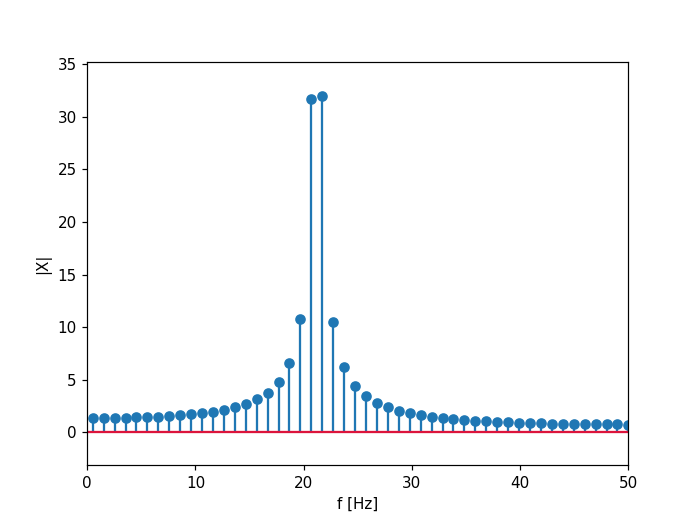interactive(children=(FloatSlider(value=20.3, description='f0', max=22.0, min=20.0, step=0.01), Output()), _do…


Quadratic or parabolic interpolation can be used to estimate the true peak of the sinusoid. using the detected maximum $$a$$ and its upper and lower frequency bin.

$$p = 0.5 * (\alpha-\gamma)/(\alpha-2*\beta+\gamma)$$

$$a^* = \beta-1/4*(\alpha-\gamma)*$$

Details on JOS Website

# Additive & Spectral: Partial Tracking

Partial tracking is the process of detecting single sinusoidal components in a signal and obtaining their individual amplitude, freuquency and phase trajectories.

## Monophonic

• STFT

A short term fourier transform segments a signal into frames of equal length.

• Fundamental Frequency Estimation - YIN (de Cheveigné et al, 2002) - Swipe (Camacho, 2007)

• Peak Detection

For every STFT frame, local maxima are calculated in the range of integer multiples of the fundamental frequency.Trajectories of partial amplitudes for a violin sound.Trajectories of partial frequencies for a violin sound.Trajectories of unwrapped partial phases for a violin sound.

# Additive & Spectral: Spectral Modeling

## McAulay/Quatieri

Sinusoidal modeling can be conisdered a higher level algorithm for the additive synthesis of harmonic sounds. It has first been used in speech processing by McAulay, R. and Quatieri (1986). For low framerates they proposed a time-domain method for partial synthesis with original phases of the partials.R. McAulay and T. Quatieri (1986)

## SMS

The above presented sinusoidal modeling approach captures only the harmonic portion of a sound. With the Sinusoids plus Noise model (SMS), Serra and Smith (1990) introduced the Deterministic + Stochastic model for spectral modeling, in order to model components in the signal which are not captured by partial tracking. A sound is therefor modeled as a combination of a dererministic component - the sinusoids - and a stochasctic component:

$$x = x_{DET} + x_{STO}$$Deterministic + Stochastic model (Serra and Smith, 1990)

## Sines + Transients + Noise

Even the harmonic and noise model can not capture all components of musical sounds. The third - and in this line last - signal component to be included are the transients.Sines + Transients + Noise (Levine and Smith, 1998)

• Arturo Camacho. Swipe: A Sawtooth Waveform Inspired Pitch Estimator for Speech and Music. PhD thesis, University of Florida, Gainesville, FL, USA, 2007.
[BibTeX▼]
• Julius O. Smith and Xavier Serra. PARSHL: An Analysis/Synthesis Program for Non-Harmonic Sounds Based on a Sinusoidal Representation. In Proceedings of the International Computer Music Conference (ICMC). Barcelona, Spain, 2005. URL: http://www.bibsonomy.org/bibtex/2fa00c44d6ff2549f4d0559a105631fd7/zazi.
[BibTeX▼]
• Alain de Cheveigné and Hideki Kawahara. YIN, a Fundamental Frequency Estimator for Speech and Music. The Journal of the Acoustical Society of America, 111(4):1917–1930, 2002.
[BibTeX▼]
• Scott Levine and Julius Smith. A Sines + Transients + Noise Audio Representation for Data Compression and Time/Pitch Scale Modifications. In Proceedings of the 105th Audio Engineering Society Convention. San Francisco, CA, 1998.
[BibTeX▼]
• Xavier Serra and Julius Smith. Spectral Modeling Synthesis: A Sound Analysis/Synthesis System Based on a Deterministic Plus Stochastic Decomposition . Computer Music Journal, 14(4):12–14, 1990.
[BibTeX▼]
• R. McAulay and T. Quatieri. Speech analysis/Synthesis based on a sinusoidal representation. Acoustics, Speech and Signal Processing, IEEE Transactions on, 34(4):744–754, 1986.
[BibTeX▼]
• T Quatieri and Rl McAulay. Speech transformations based on a sinusoidal representation. IEEE Transactions on Acoustics, Speech, and Signal Processing, 34(6):1449–1464, 1986.
[BibTeX▼]
• # Additive & Spectral: Fourier Series

## Basic Waveforms

Well known basic waveforms can be generated with spefific Fourier series. This knowledge can be used to synthesize musical timbres, since the sound of certain instruments is similar to these basic waveforms.

The following examples illustrate properties of spectra and waveforms for three basic waveforms. In a Jupyter notebook, fundamental frequency and number of partials can be adjusted and the result can be played back. In the static HTML version, fixed values are used.

### Triangular

• only odd harmonics
• alternating sign (phase)

$$X(t) = \frac{8}{\pi^2} \sum\limits_{i=0}^{N} (-1)^{(i)} \frac{\sin(2 \pi (2i +1) f\ t)}{(2i +1)^2}$$

# define a Python function for the triangular wave

def triang(t,f0, fs, nPartials):

y = np.zeros(len(t))
for partCNT in range(nPartials):
if partCNT*f0 < fs/4:
y += (8/pow(pi,2)) * pow(-1, partCNT) * \
sin(2*pi* f0 * (2* partCNT +1) *t) *  \
(1/pow(2*partCNT+1,2))

return y

# visualize spectrum

import numpy as np
from   numpy import linspace, sin, zeros
from   math import pi
%matplotlib notebook
import matplotlib.pyplot as plt
from   tikzplotlib import save as tikz_save

from   IPython.display import display, Markdown, clear_output
import IPython.display as ipd
import ipywidgets as widgets
from   ipywidgets import *

nPartials   = 10 # number of partials
f0          = 200  # signal frequency
fs          = 48000
N           = fs

t = np.linspace(0,N/fs,N)
f = np.linspace(-0.5,0.5,fs)

x = triang(t,f0,fs,nPartials)

fig = plt.figure()
plt.title("Triangular")

X = abs(np.fft.fft(x))
X = np.fft.fftshift(X)
f = np.linspace(-fs/2,fs/2,len(X))

line, = ax.plot(f,X);

ax.set_xlabel('f [Hz]');
ax.set_ylabel('|X|');
ax.set_xlim(0, fs/2)

print('Static output:')
ipd.display(ipd.Audio(x, rate=fs))
print('Dynamic output:')

def update(nPartials = widgets.IntSlider(min = 0, max= 100, step=1, value=5),
f0 = widgets.IntSlider(min = 1, max= 1000, step=1, value=200)):

x = triang(t,f0,fs,nPartials)

X = abs(np.fft.fft(x))
X = np.fft.fftshift(X)

line.set_ydata(X)
fig.canvas.draw_idle()

ipd.display(ipd.Audio(x, rate=fs))

interact(update);

<IPython.core.display.Javascript object>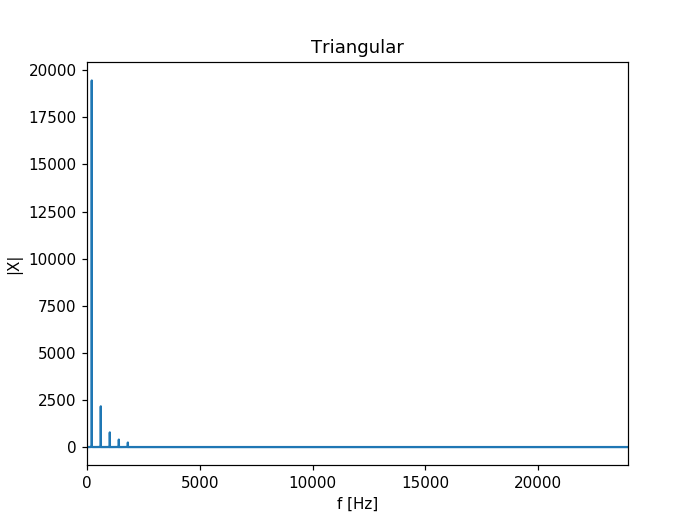Static output:

Dynamic output:

interactive(children=(IntSlider(value=5, description='nPartials'), IntSlider(value=200, description='f0', max=…


### Square Wave

• only odd harmonics
• constant sign
• found in spectra of wind instruments

$$X(t) = \frac{4}{\pi} \sum\limits_{i=0}^{N} \frac{\sin(2 \pi (2i+1)ft)}{(2i + 1)}$$

# define a Python function for the square wave

def square(t,f0, fs, nPartials):

y = np.zeros(len(t))

for partCNT in range(nPartials):

if partCNT*f0 < fs/4:
y += (4/np.pi) * (np.sin(2*np.pi* f0 * (2* partCNT +1) *t)/(2*partCNT+1))

return y

# visualize spectrum

nPartials   = 10 # number of partials
f0          = 200  # signal frequency
fs          = 48000
N           = fs

t = np.linspace(0,N/fs,N)
f = np.linspace(-0.5,0.5,fs)

x = square(t,f0,fs,nPartials)

fig = plt.figure()
plt.title("Square")

X = abs(np.fft.fft(x))
X = np.fft.fftshift(X)
f = np.linspace(-fs/2,fs/2,len(X))

line, = ax.plot(f,X);

ax.set_xlabel('f [Hz]');
ax.set_ylabel('|X|');
ax.set_xlim(0, fs/2)

print('Static output:')
ipd.display(ipd.Audio(x, rate=fs))
print('Dynamic output:')

def update(nPartials = widgets.IntSlider(min = 0, max= 100, step=1, value=10),
f0 = widgets.IntSlider(min = 1, max= 1000, step=1, value=200)):

x = square(t,f0,fs,nPartials)

X = abs(np.fft.fft(x))
X = np.fft.fftshift(X)

line.set_ydata(X)
fig.canvas.draw_idle()

ipd.display(ipd.Audio(x, rate=fs))

interact(update);

<IPython.core.display.Javascript object>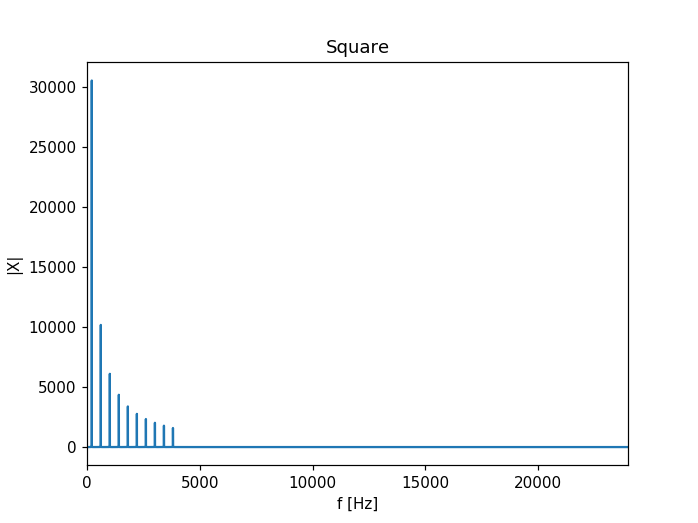Static output:

Dynamic output:

interactive(children=(IntSlider(value=10, description='nPartials'), IntSlider(value=200, description='f0', max…


### Sawtooth

• odd and even harmonics
• alternating sign

$$X(t) = \frac{2}{\pi} \sum\limits_{k=1}^{N} (-1)^i \frac{\sin(2 \pi i f\ t)}{i}$$

# define a Python function for the sawtooth

def sawtooth(t,f0, fs, nPartials):

y = np.zeros(len(t))

for partCNT in range(nPartials-1):

if partCNT*f0 < fs/2:

y += (2/np.pi) * pow(-1,(partCNT+1)) * (np.sin(2*np.pi* f0 * (partCNT +1) *t)/(partCNT+1))

return y

# visualize spectrum

nPartials   = 10 # number of partials
f0          = 200  # signal frequency
fs          = 48000
N           = fs

t = np.linspace(0,N/fs,N)
f = np.linspace(-0.5,0.5,fs)

x = sawtooth(t,f0,fs,nPartials)

fig = plt.figure()
plt.title("Sawtooth")

X = abs(np.fft.fft(x))
X = np.fft.fftshift(X)
f = np.linspace(-fs/2,fs/2,len(X))

line, = ax.plot(f,X);

ax.set_xlabel('f [Hz]');
ax.set_ylabel('|X|');
ax.set_xlim(0, fs/2)

print('Static output:')
ipd.display(ipd.Audio(x, rate=fs))
print('Dynamic output:')

def update(nPartials = widgets.IntSlider(min = 0, max= 100, step=1, value=10),
f0 = widgets.IntSlider(min = 1, max= 1000, step=1, value=200)):

x = sawtooth(t,f0,fs,nPartials)

X = abs(np.fft.fft(x))
X = np.fft.fftshift(X)

line.set_ydata(X)
fig.canvas.draw_idle()

ipd.display(ipd.Audio(x, rate=fs))

interact(update);

<IPython.core.display.Javascript object>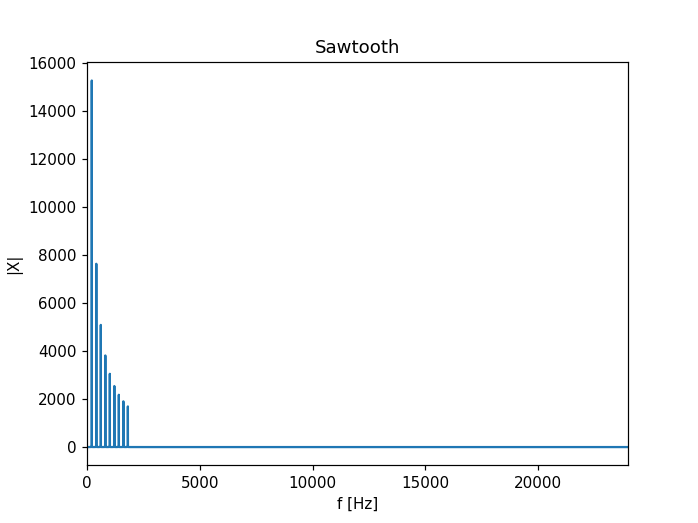Static output:

Dynamic output:

interactive(children=(IntSlider(value=10, description='nPartials'), IntSlider(value=200, description='f0', max…


## Time Domain

### Triangular

nPartials   = 5 # number of partials
f0          = 200  # signal frequency
fs          = 48000
N           = fs

t = np.linspace(0,N/fs,N)
x = triang(t,f0,fs,nPartials)

fig = plt.figure()
plt.title("Triangular")

line, = ax.plot(t,x);

ax.set_xlabel('t [s]');
ax.set_ylabel('|X|');
ax.set_xlim(0, 0.025)

print('Static output:')
ipd.display(ipd.Audio(x, rate=fs))
print('Dynamic output:')

def update(nPartials = widgets.IntSlider(min = 0, max= 100, step=1, value=5),
f0 = widgets.IntSlider(min = 1, max= 1000, step=1, value=200)):

x = triang(t,f0,fs,nPartials)

line.set_ydata(x)
fig.canvas.draw_idle()

ipd.display(ipd.Audio(x, rate=fs))

interact(update);

<IPython.core.display.Javascript object>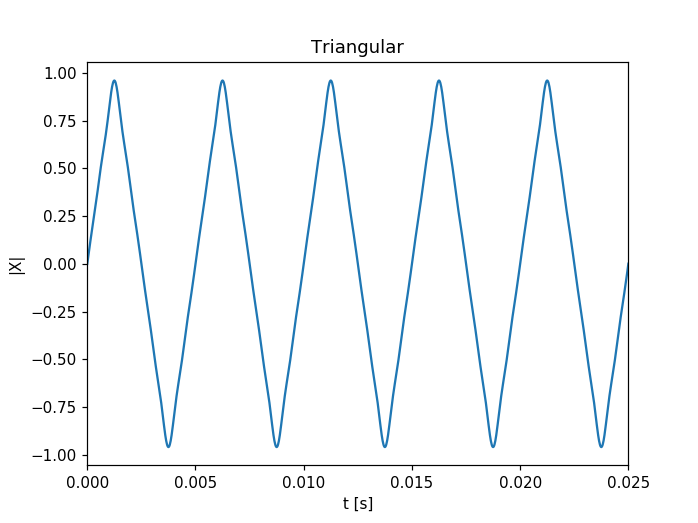Static output:

Dynamic output:

interactive(children=(IntSlider(value=5, description='nPartials'), IntSlider(value=200, description='f0', max=…


## Square

For the square wave, ripples occur at the edges, referred to as Gibb's phenomenon.

nPartials   = 5 # number of partials
f0          = 200  # signal frequency
fs          = 48000
N           = fs

t = np.linspace(0,N/fs,N)
x = square(t,f0,fs,nPartials)

fig = plt.figure()
plt.title("Square")

line, = ax.plot(t,x);

ax.set_xlabel('t [s]');
ax.set_ylabel('|X|');
ax.set_xlim(0, 0.025)

print('Static output:')
ipd.display(ipd.Audio(x, rate=fs))
print('Dynamic output:')

def update(nPartials = widgets.IntSlider(min = 0, max= 100, step=1, value=5),
f0 = widgets.IntSlider(min = 1, max= 1000, step=1, value=200)):

x = square(t,f0,fs,nPartials)

line.set_ydata(x)
fig.canvas.draw_idle()

ipd.display(ipd.Audio(x, rate=fs))

interact(update);

<IPython.core.display.Javascript object>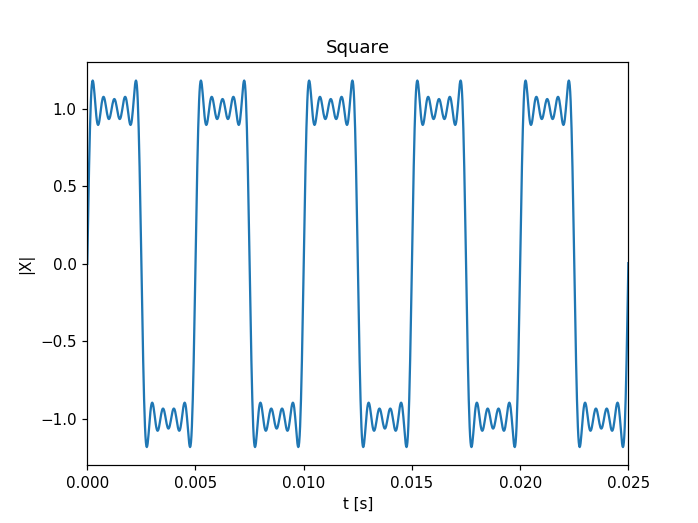Static output:

Dynamic output:

interactive(children=(IntSlider(value=5, description='nPartials'), IntSlider(value=200, description='f0', max=…


## Sawtooth

nPartials   = 5 # number of partials
f0          = 200  # signal frequency
fs          = 48000
N           = fs

t = np.linspace(0,N/fs,N)
x = sawtooth(t,f0,fs,nPartials)

fig = plt.figure()
plt.title("Sawtooth")

line, = ax.plot(t,x);

ax.set_xlabel('t[s]');
ax.set_ylabel('|X|');
ax.set_xlim(0, 0.025)

print('Static output:')
ipd.display(ipd.Audio(x, rate=fs))
print('Dynamic output:')

def update(nPartials = widgets.IntSlider(min = 0, max= 100, step=1, value=5),
f0 = widgets.IntSlider(min = 1, max= 1000, step=1, value=200)):

x = sawtooth(t,f0,fs,nPartials)

line.set_ydata(x)
fig.canvas.draw_idle()

ipd.display(ipd.Audio(x, rate=fs))

interact(update);

<IPython.core.display.Javascript object>